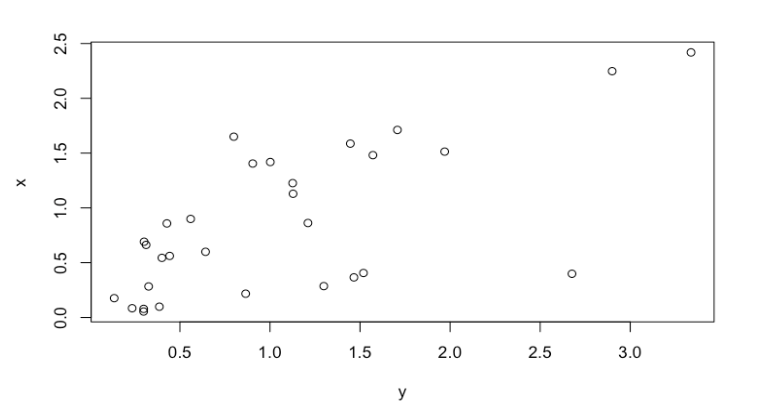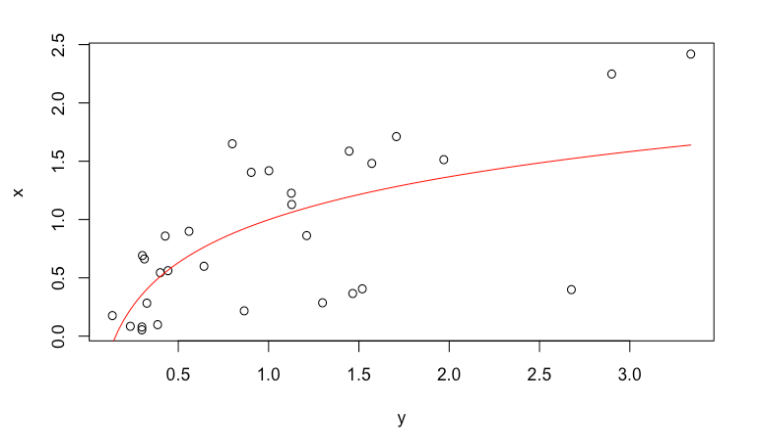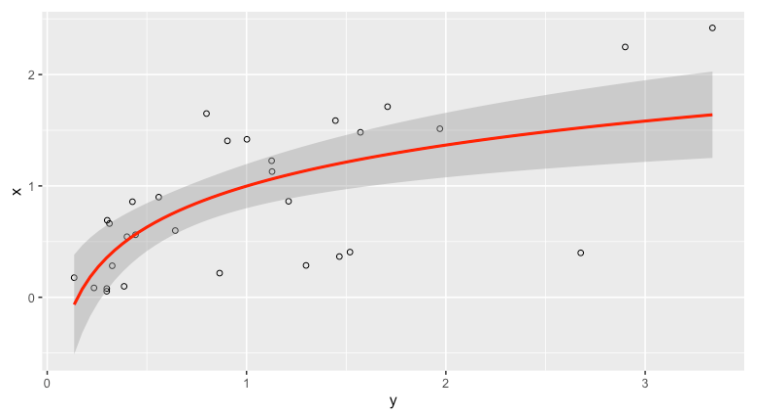# Fit Logarithmic Curve in R (2 Examples)

In this article you’ll learn how to fit a logarithmic curve in a plot in the R programming language.

The table of content is structured as follows:

Let’s see how this can be done.

## Example Data & Basic Graphic

We will use the following data as a basis for this tutorial:

```set.seed(4353266)
x <- abs(rnorm(30))
y <- abs(rnorm(30) + 0.3 * x^3)
data <- data.frame(x,y)As shown, our data consists of two numerical columns. We will also need to create a linear regression model using the lm() function. This function allows us to fit a linear model to our data frame:

```lm_log <- lm(x~log(y),
data)```

Now, we can draw our data:

```plot(x~y,
data)```## Example 1: Fit Logarithmic Curve in Graph Using curve() Function

The curve() function right after our plot can help us to fit a logarithmic curve in a graphic. Let’s see how it works:

```plot(x~y,
data)
curve(coef(lm_log) +
coef(lm_log)*log(x),
col = "red")```## Example 2: Fit Logarithmic Curve in Graph Using ggplot2

In order to draw our data, please install the ggplot2 package if you haven’t installed it before:

`install.packages("ggplot2")`

`library(ggplot2)`

Next, we can use the ggplot and geom_point functions to draw a ggplot scatterplot and the stat_smooth function to add a fitted logarithmic line on top of this plot:

```ggplot(data,
aes(y,x)) +
geom_point(shape = 1) +
stat_smooth(method = "lm",
formula = y ~ log(x),
col = "red")```As you can see, it is possible to draw a logarithmic curve in a plot both with the curve() function and the ggplot2 package.

## Video, Further Resources & Summary

Do you need more explanations on how to draw a logarithmic curve in a graph? Then you should have a look at the following YouTube video of the Statistics Globe YouTube channel.

Furthermore, you could have a look at some of the other tutorials on Statistics Globe:

This post has shown how to add a logarithmic curve to a graphic in R. In case you have further questions, you might leave a comment below.